Updating search results...

# 21 Results

View
Selected filters: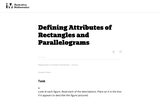Unrestricted Use
CC BY
Rating
3.0 stars
Overview:

This is a task from the Illustrative Mathematics website that is one part of a complete illustration of the standard to which it is aligned. Each task has at least one solution and some commentary that addresses important asects of the task and its potential use. Here are the first few lines of the commentary for this task: Look at each figure. Read each of the descriptions. Place an X in the box if it appears to describe the figure pictured. A. B. C. D. 4 vertices Four si...

Subject:
Mathematics
Material Type:
Activity/Lab
Provider:
Illustrative Mathematics
Provider Set:
Illustrative Mathematics
Author:
Illustrative Mathematics
03/30/2014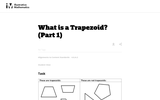Unrestricted Use
CC BY
Rating
2.0 stars
Overview:

This is a task from the Illustrative Mathematics website that is one part of a complete illustration of the standard to which it is aligned. Each task has at least one solution and some commentary that addresses important asects of the task and its potential use. Here are the first few lines of the commentary for this task: Say what a trapezoid is in your own words. Compare your definition with a partner. Is this parallelogram a trapezoid according to your definition? Expl...

Subject:
Mathematics
Material Type:
Activity/Lab
Provider:
Illustrative Mathematics
Provider Set:
Illustrative Mathematics
Author:
Illustrative Mathematics
05/20/2013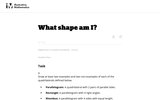Unrestricted Use
CC BY
Rating
3.5 stars
Overview:

This is a task from the Illustrative Mathematics website that is one part of a complete illustration of the standard to which it is aligned. Each task has at least one solution and some commentary that addresses important asects of the task and its potential use. Here are the first few lines of the commentary for this task: Draw at least two examples and two non-examples of each of the quadrilaterals defined below. Parallelogram: A quadrilateral with 2 pairs of parallel si...

Subject:
Mathematics
Material Type:
Activity/Lab
Provider:
Illustrative Mathematics
Provider Set:
Illustrative Mathematics
Author:
Illustrative Mathematics
05/16/2013Conditional Remix & Share Permitted
CC BY-NC-SA
Rating
0.0 stars
Overview:

This book is a "flexed" version of CK-12's Basic Geometry that aligns with College Access Geometry and contains embedded literacy supports. It covers the essentials of geometry for the high school student.

Subject:
Mathematics
Material Type:
Textbook
Provider:
CK-12 Foundation
Provider Set:
CK-12 FlexBook
Author:
Fauteux, Michael
Zapata, Rosamaria
05/18/2011Conditional Remix & Share Permitted
CC BY-NC-SA
Rating
4.0 stars
Overview:

CK-12 Foundation's Geometry FlexBook is a clear presentation of the essentials of geometry for the high school student. Topics include: Proof, Congruent Triangles, Quadrilaterals, Similarity, Perimeter & Area, Volume, and Transformations.

Subject:
Mathematics
Material Type:
Textbook
Provider:
CK-12 Foundation
Provider Set:
CK-12 FlexBook
10/06/2009Conditional Remix & Share Permitted
CC BY-NC-SA
Rating
0.0 stars
Overview:

A structured geometry program teacher edition of daily lesson plans and teacher supports to accompany the College Access Reader: Geometry student edition.

Subject:
Mathematics
Material Type:
Textbook
Provider:
CK-12 Foundation
Provider Set:
CK-12 FlexBook
Author:
Fauteux, Michael
Zapata, Rosamaria
05/18/2011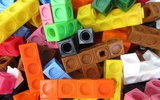Conditional Remix & Share Permitted
CC BY-NC-SA
Rating
0.0 stars
Overview:

In this lesson students will explore characteristics of quadrilaterals.

Subject:
Mathematics
Material Type:
Formative Assessment
Author:
Drew Polly
07/27/2020Rating
3.0 stars
Overview:

Students use geoboards to make a quadrilateral. Students copy their quadrilateral onto geoboard paper and record as many different quadrilaterals as they can and tell how the quadriaterals are alike and different.

Subject:
Mathematics
Material Type:
Activity/Lab
Provider:
K-5 Math Teaching Resources
Author:
K-5 Math Teaching Resources
02/26/2019Conditional Remix & Share Permitted
CC BY-NC-SA
Rating
0.0 stars
Overview:

CK-12 Foundation's Geometry FlexBook is a clear presentation of the essentials of geometry for the high school student. Topics include: Proof, Congruent Triangles, Quadrilaterals, Similarity, Perimeter & Area, Volume, and Transformations.

Subject:
Mathematics
Material Type:
Textbook
Provider:
CK-12 Foundation
Provider Set:
CK-12 FlexBook
11/23/2010Conditional Remix & Share Permitted
CC BY-NC-SA
Rating
0.0 stars
Overview:

CK-12's Geometry - Second Edition is a clear presentation of the essentials of geometry for the high school student. Topics include: Proofs, Triangles, Quadrilaterals, Similarity, Perimeter & Area, Volume, and Transformations.

Subject:
Mathematics
Material Type:
Textbook
Provider:
CK-12 Foundation
Provider Set:
CK-12 FlexBook
Author:
Dirga, Kathryn
Jordan, Lori
11/16/2010Conditional Remix & Share Permitted
CC BY-NC-SA
Rating
0.0 stars
Overview:

CK-12 Geometry Teacher's Edition covers tips, common errors, enrichment, differentiated instruction and problem solving for teaching CK-12 Geometry Student Edition. The solution and assessment guides are available upon request.

Subject:
Mathematics
Material Type:
Textbook
Provider:
CK-12 Foundation
Provider Set:
CK-12 FlexBook
Author:
Kershaw, Jem
Kramer, Melissa
Zwack, Teresa
06/25/2011Conditional Remix & Share Permitted
CC BY-NC-SA
Rating
0.0 stars
Overview:

This document is from NC2ML and state leaders. It provides an overview for teachers about the mathematics and pedagogies in Grade 3 Cluster 5. This is not remixable since it is from state leaders.

Subject:
Mathematics
Material Type:
Reference Material
Author:
Drew Polly
11/16/2019Conditional Remix & Share Permitted
CC BY-NC-SA
Rating
0.0 stars
Overview:

In this lesson, students explore various quadrilaterals by sorting them based on attributes such as opposite sides having equal lengths and square corners (right angles).

Subject:
Mathematics
Material Type:
Activity/Lab
Lesson Plan
Author:
Drew Polly
11/18/2019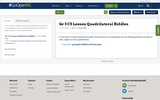Conditional Remix & Share Permitted
CC BY-NC-SA
Rating
5.0 stars
Overview:

In this lesson, students identify and explain the attributes of a quadrilateral and use defining attributes (number of sides, angles) to name quadrilaterals.

Subject:
Mathematics
Material Type:
Activity/Lab
Lesson Plan
Author:
Drew Polly
11/18/2019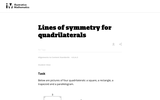Unrestricted Use
CC BY
Rating
0.0 stars
Overview:

This task provides students a chance to experiment with reflections of the plane and their impact on specific types of quadrilaterals. It is both interesting and important that these types of quadrilaterals can be distinguished by their lines of symmetry.

Subject:
Mathematics
Material Type:
Activity/Lab
Provider:
Illustrative Mathematics
Provider Set:
Illustrative Mathematics
Author:
Illustrative Mathematics
11/11/2012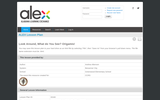Rating
0.0 stars
Overview:

Students will be able to identify polygons or shapes in the world around them and use those polygons to build origamis. Students will reinforce their understanding and play a geometric computer game when they have finished.

Subject:
Mathematics
Material Type:
Lesson Plan
Provider:
Alabama Learning Exchange
Author:
Andrea Aikerson
02/26/2019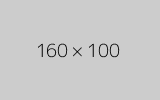Conditional Remix & Share Permitted
CC BY-NC
Rating
0.0 stars
Overview:

Four full-year digital course, built from the ground up and fully-aligned to the Common Core State Standards, for 7th grade Mathematics. Created using research-based approaches to teaching and learning, the Open Access Common Core Course for Mathematics is designed with student-centered learning in mind, including activities for students to develop valuable 21st century skills and academic mindset.

Subject:
Mathematics
Material Type:
Full Course
Provider:
Pearson
11/02/2020Rating
0.0 stars
Overview:

Constructions and Angles

Unit Overview

Type of Unit: Concept

Prior Knowledge

Students should be able to:

Use a protractor and ruler.
Identify different types of triangles and quadrilaterals and their characteristics.

Lesson Flow

After an initial exploratory lesson involving a paper folding activity that gets students thinking in general about angles and figures in a context, the unit is divided into two concept development sections. The first section focuses on types of angles—adjacent, supplementary, complementary, and vertical—and how they are manifested in quadrilaterals. The second section looks at triangles and their properties, including the angle sum, and how this affects other figures.

In the first set of conceptual lessons, students explore different types of angles and where the types of angles appear in quadrilaterals. Students fold paper and observe the angles formed, draw given angles, and explore interactive sketches that test many cases. Students use a protractor and ruler to draw parallelograms with given properties. They explore sketches of parallelograms with specific properties, such as perpendicular diagonals. After concluding the investigation of the angle types, students move on to the next set.

In the second set of conceptual development lessons, students focus on triangles. Students again fold paper to create figures and certain angles, such as complementary angles.

Students draw, using a protractor and ruler, other triangles with given properties. Students then explore triangles with certain known and unknown elements, such as the number of given sides and angles. This process starts with paper folding and drawing and continues with exploration of interactive sketches. Students draw conclusions about which cases allow 0, 1, 2, or an infinite number of triangles. In the course of the exploration, students discover that the sum of the measure of the interior angles of a triangle is 180°. They also learn that the sum of the measures of the interior angles of a quadrilateral is 360°. They explore other polygons to find their angle sum and determine if there is a relationship to angle sum of triangles. The exploration concludes with finding the measure of the interior angles of regular polygons and speculating about how this relates to a circle.

Lastly, students solve equations to find unknown angle measures. Using their previous experience, students find the remaining angle measures in a parallelogram when only one angle measure is given. Students also play a game similar to 20 Questions to identify types of triangles and quadrilaterals. Having completed the remaining lessons, students have a four-day Gallery to explore a variety of problems.

The unit ends with a unit assessment.

Subject:
Mathematics
Geometry
Provider:
Pearson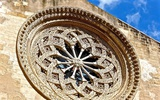Conditional Remix & Share Permitted
CC BY-NC
Rating
0.0 stars
Overview:

Lesson OverviewStudents explore different polygons by drawing them, measuring angles, and manipulating interactive sketches to find the angle sum for any polygon. Students also explore the angle measures in regular polygons.Key ConceptsThe angle sum in a triangle is 180°. A quadrilateral can be composed of two triangles, so the angle sum of a quadrilateral is 360°.The number of triangles that compose a polygon is two less than the number of sides (angles). The sum of the interior angles in a polygon with n sides is 180° (n – 2).Goals and Learning ObjectivesFind angle sums in polygons.Generalize to find the angle sum for any polygon.Find interior angle measures for regular polygons.

Subject:
Geometry
Material Type:
Lesson Plan
Author:
Pearson
11/02/2020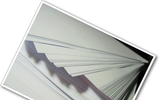Conditional Remix & Share Permitted
CC BY-NC
Rating
0.0 stars
Overview:

Gallery OverviewAllow students who have a clear understanding of the content thus far in the unit to work on Gallery problems of their choosing. You can then use this time to provide additional help to students who need review of the unit’s concepts or to assist students who may have fallen behind on work.Problem DescriptionsParallelogram to CubeStudents have a chance to review angle measurements in a parallelogram. Building the cube helps students see the transition from two-dimensional shapes and their relationship to three-dimensional figures.QuadrilateralsStudents investigate the possible quadrilaterals that can be made from any four given side lengths, focusing on those that can’t make a quadrilateral. Students also look at possible parallelograms with two sides given and possible rhombuses with four sides given.DiagonalsStudents further investigate diagonals in quadrilaterals. If the diagonals are perpendicular, is the figure a rhombus?TrapezoidsHow many right angles can a trapezoid have? How many congruent angles or congruent sides can it have? Can its diagonals be perpendicular or congruent? Students investigate possible trapezoids.More AnglesStudents explore three intersecting lines and the combinations of angles.Diagonals and AnglesThe sides of a parallelogram are extended beyond the vertices, and students explore which angles are congruent and which are supplementary. Students also explore the effect diagonals have on interior angles.Exterior AnglesStudents  explore the sum of exterior angles for several polygons and speculate about the results.Angles and SidesStudents explore the relationship between angles and sides in a triangle and discover that the longest side is opposite the largest angle, and the shortest side is opposite the smallest angle (and congruent sides are opposite congruent angles).Ratios and AnglesStudents explore the ratios of the legs of a right triangle to the angles in the triangle. Students see that there is a unique ratio for each angle, and vice versa. This is an informal look at trigonometry.Find the AngleStudents solve equations to find angle measures in polygons.TessellationsStudents explore quadrilateral tessellations and why they tessellate. Students also explore tessellations of pentagons and other polygons.

Subject:
Geometry
Material Type:
Lesson Plan
Author:
Pearson